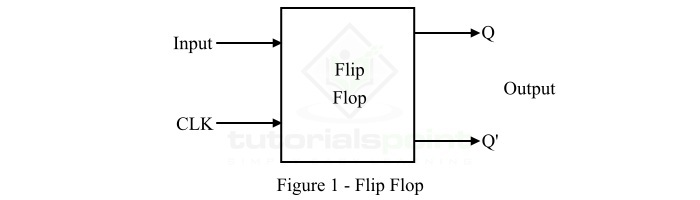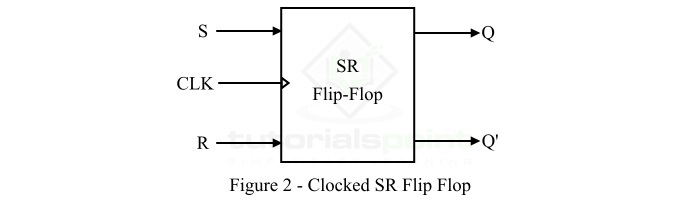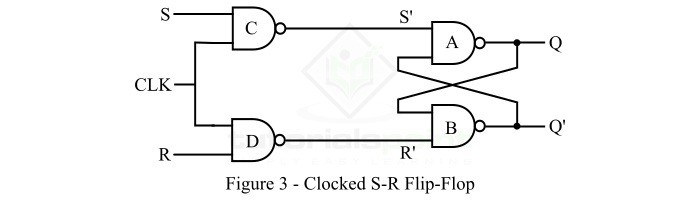# Synchronous or Clocked S-R Flip-Flop

In digital electronics, a flip-flop is a most elementary memory element used in several electronic circuit to store 1-bit information. A flip-flop is a basically a bistable multivibrator having two stable states.

Flip-flops are made up of an interconnection of logic gates. However, a logic gate itself does not storage capability, but when several logic gates are arranged in a specific manner, they can store information. Also, flip-flop is the most fundamental building block of sequential logic circuits. The block diagram representation of a typical flip-flop is shown in Figure-1.A flip-flop has one or more inputs and two outputs, usually represented by Q and Q' along with a clock input. The clock input is used to trigger the flip-flop so that it can change states of its outputs.

There are several types of flip-flops available such as S-R flip-flop, J-K flip-flop, D flip-flop, T flip-flop. Each type of flip-flop has its unique features and characteristics required for a particular application.

## Synchronous and Asynchronous Flip-flops

A flip-flop whose logic circuit is clocked/triggered by a clock signal is known as a synchronous flip-flop. Thus, the output states of the synchronous flip-flop do not change in the absence of the clock signal, even if its inputs change many times.

On the other hand, an asynchronous flip-flop is one in which there is no clock signal, hence its output changes instantly on the application of inputs.

Now, let us discuss the clocked or synchronous S-R flip-flop in detail.

## What is a Clocked SR Flip-flop?

The type flip-flop which has two inputs namely S (Set) and R (Reset) is termed as an SR flipflop. If the S and R inputs of the flip-flop control its outputs when a clock pulse is present (i.e. goes from either low to high or high to low), then it called a clocked SR flip-flop. Since, the clock signal synchronizes the operation of the SR flip-flop, hence the clocked SR flip-flop is also known as synchronous SR flip-flop. The block diagram of a clocked or synchronous S-R flip-flop is shown in Figure-2.The logic circuit diagram of the clocked or synchronous SR flip-flop is shown in Figure-3 below.As it can be seen that the circuit consists of four NAND gates. The clock signal is connected to the NAND gates C and D and the inputs S and R also applied to the NAND gates C and D. The NAND gates A and B are cross-coupled to form the storage circuit of the flip-flop.

## Operation of Clocked SR Flip-Flop

The operation of this circuit of clocked SR flip-flop is as described as follows −

• When the clock signal is not applied, the SR flip-flop circuit remains inactive, and there is no change in the outputs of the flip-flop.

• When the clock signal is applied, the flip-flop circuit becomes active and operates as explained below −

• When S = 0 and R = 0, the output of NAND gates C and D are S' = 1 and R' = 1. Hence, the outputs of the NAND gates A and B remains unchanged. This is called Hold State of the SR flip-flop.

• When S = 0 and R = 1, the output of the NAND gates C and D are S' = 1 and R' = 0, the output of the NAND gate A is 0 and that of NAND gate B is 1. This is called Reset State of the SR flip-flop.

• When S = 1 and R = 0, the output of the NAND gates C and D are S' = 0 and R' = 1, the output of the NAND gate A is 1 and that of the NAND gate B is 0. This is called Set State of the SR flip-flop.

• When S = 1 and R = 1, the output of the NAND gates C and D are S' = 0 and R' = 0, the outputs of the both NAND gates A and B try to become 1, which is not possible. This is called Forbidden State of the SR flip-flop.

We can also express the operation of the clocked SR flip-flop in the form of a truth table as given below. Here, S and R specifies the inputs, Qn specifies the present state of the output, and Qn+1 specifies the state of the output after change in input and application of clock pulse.

Inputs Output Comment
SRQnQn+1
0000No Change / Hold
0011No Change / Hold
0100Reset
0110Reset
1001Set
1011Set
110XForbidden
111XForbidden

From this truth table of the clocked SR flip-flop we can directly write the Boolean expression for its output Qn+1 as follows −Hence, the characteristics equation of the SR flip-flop is,

$$Q_{n+1}=S+R'Q_{n}$$

## Applications of Clocked SR Flip-Flop

The clocked SR flip-flops are used in the following applications −

• Digital counters

• Storage and shift registers

• Data storage elements

• Data transfer systems

• Frequency divider circuits, etc.

## Conclusion

A clocked SR flip-flop is a sequential logic circuit used as a 1 bit storage device in digital systems. It has two inputs S (Set) and R (Reset). When R is high, SR flip-flop is said to be in reset state; when S is high, SR flip-flop is called in set state; when both inputs S and R are high, SR flip-flop is said to be in forbidden or invalid state; and when both inputs S and R are low, SR flip-flop is said to be in no change or hold state.

Updated on: 25-Apr-2023

3K+ Views